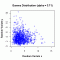•http://facebook.com/
•https://www.google.com/accounts/o8/id
•https://me.yahoo.com

# Gamma

#### CDF

Evaluates the Gamma distribution CDF.
 double CDF (double a, double b, double x, bool upper = false)

#### Random Sample

Generates random numbers following a standard gamma distribution.
 RandomSample::RandomSample unknown (double alpha, double s) RandomSample::~RandomSample unknown () void RandomSample::init (double alpha) double RandomSample::genReal () void RandomSample::Build1 (double alphax) void RandomSample::Build2 (double alphax) void RandomSample::BuildExp () double RandomSample::Gen1 () double RandomSample::Gen2 () double RandomSample::GenExp () double RandomSample::Density1 (double x) double RandomSample::Density2 (double x) double sample (double alpha, double seed=0)